### Home > A2C > Chapter 9 > Lesson 9.2.2 > Problem9-92

9-92.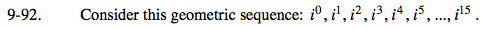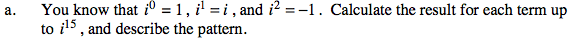i0 = 1 Can you see the pattern?

i1 = i

i2 = −1

i3 = (i)(i2) = −i

i4 = (i2)(i2) = 1

i5 = (i2)(i2)(i) = i

The pattern repeats 1, i, −1, and −i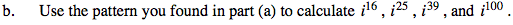16 is a multiple of 4.
25 is on more than a multiple of 4.
39 is one less than a multiple of 4.
100 is a multiple of 4.

i16 = 1
i25 = i
i39 = −i
i100 = 1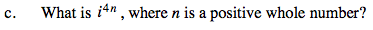4n is a multiple of 4.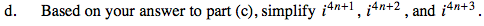Refer to (a), (b), and (c).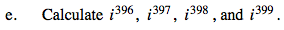i396 = i4·99 = 1

i397 = i4·99+1 = ?

i398 = i4·99+2 = ?

i399 = ?